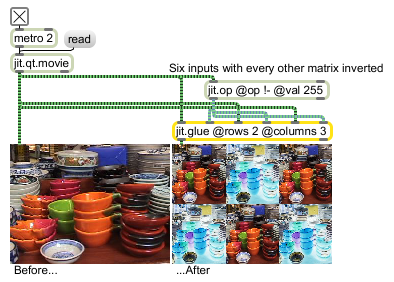# jit.glue

Glue many matrices into one

## Description

The jit.glue object takes multiple input matrices and composites them together as if they were rectangular pieces of a whole.

## Matrix Operator

matrix inputs:-1, matrix outputs:1
 Name IOProc Planelink Typelink Dimlink Plane Dim Type out n/a 1 1 0 1 1 char long float32 float64

## Attributes

Name Type g/s Description
syncinlet int The inlet number which will cause the jit.glue object to output a new composite matrix when a matrix is received at the specified inlet (default = 0)
A value of -1 will cause the object to output a new matrix in response to a matrix received at all inlets
columns int The number of columns to use when gluing the matrix together (default = 1)
If you specify the columns attribute in the object box, The number of inlets will be equal to the number of rows multiplied by the number of columns. If you change the rows * columns attributes later, the size of the split regions will change, but the number of inlets will not change.
rows int The number of rows to use when gluing the matrix together (default = 1)
If you specify the rows attribute in the object box, The number of inlets will be equal to the number of rows multiplied by the number of columns. If you change this value later you will change the compositing effect, but the number of inlets will not change.

## Examples